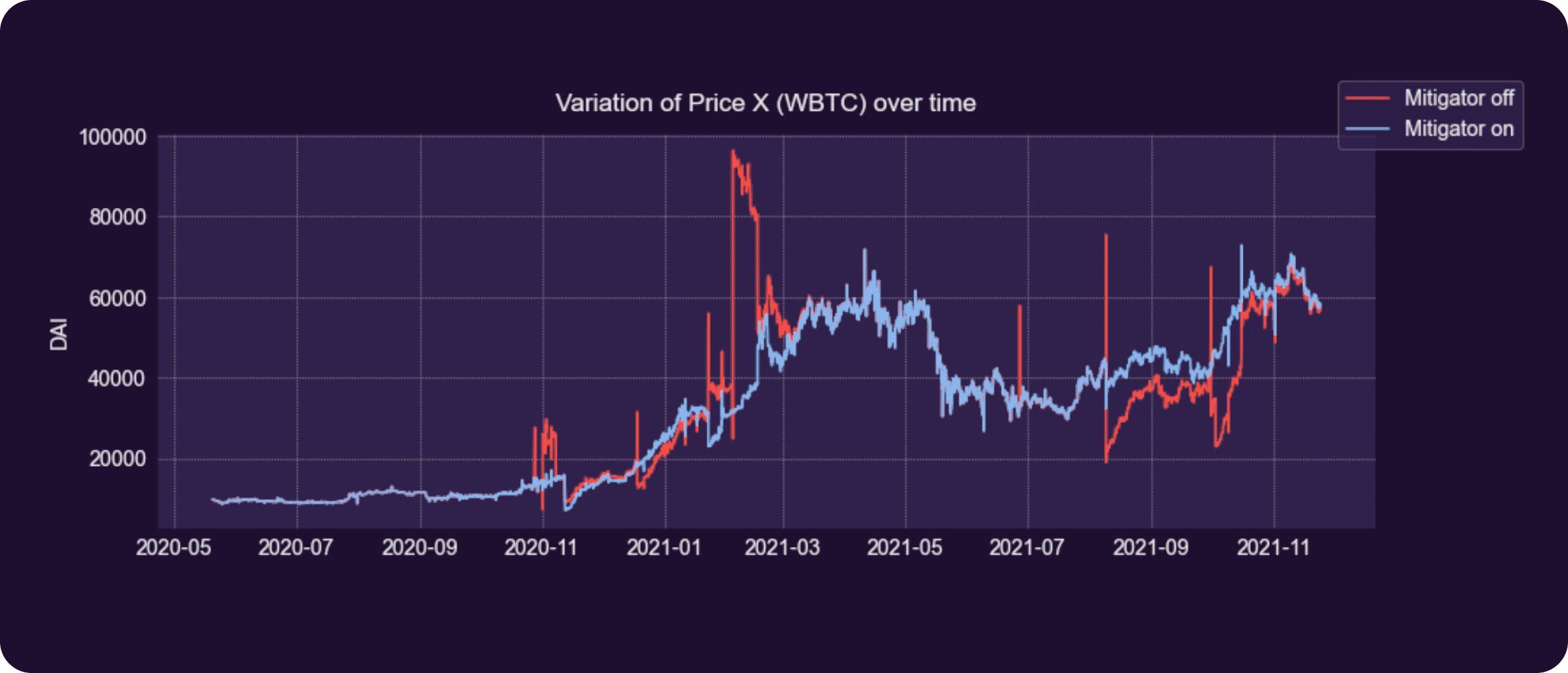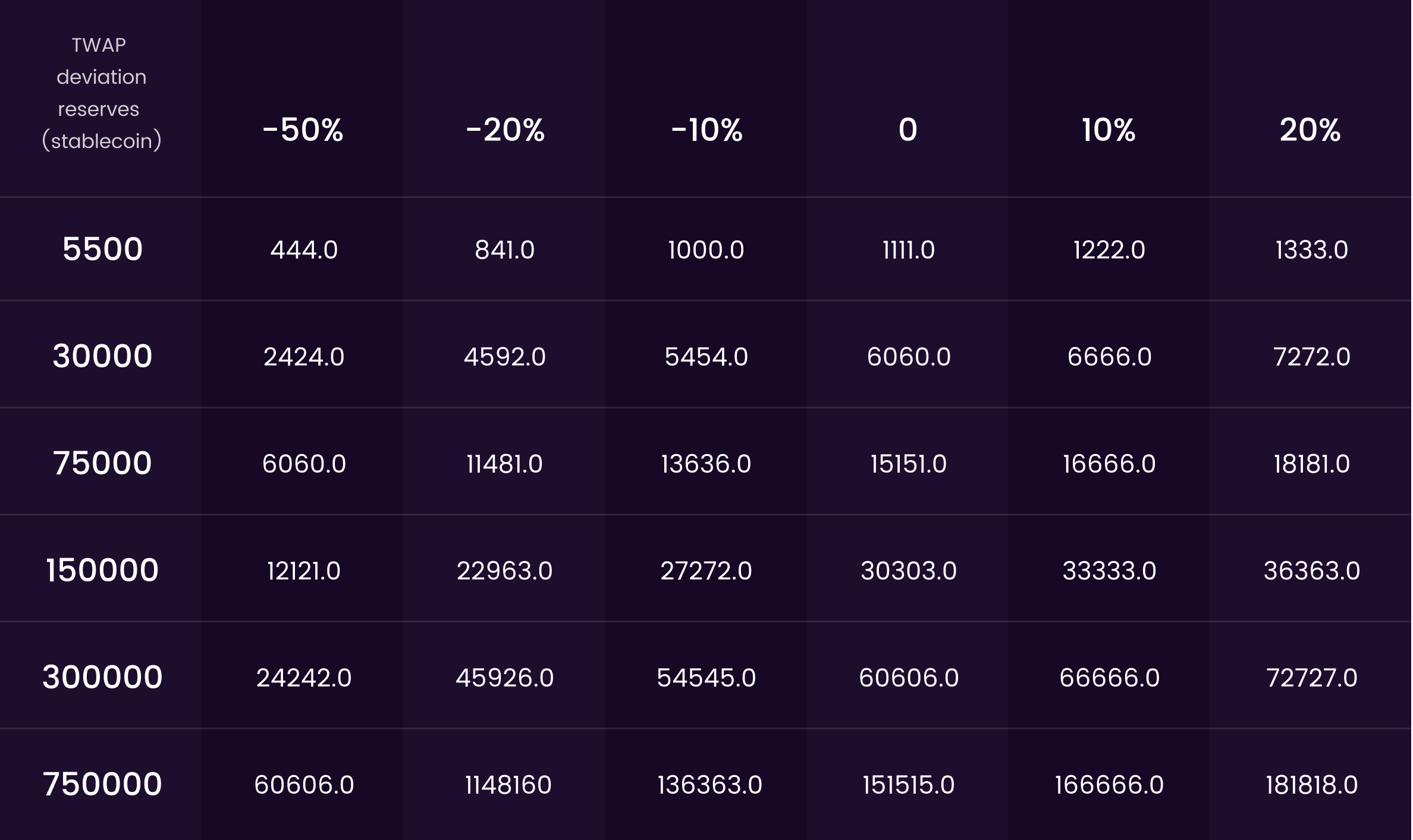By clicking “Accept”, you agree to the storing of cookies on your device to enhance site navigation, analyze site usage, and assist in our marketing efforts. View our Privacy Policy for more information.

# Volatility Mitigation Explained

In the previous article, we described the process that would allow us to simulate traders activity for subsequent conduct of AMM tests. Before proceeding further and looking into the simulation results, it’s important to acquire a basic understanding of the principles of work behind the mechanism that protects the pools from transactions that could have a detrimental impact on price stability.

This article introduces you to the main concepts you need to know about the volatility mitigation mechanism and presents a general view of how it functions. The main rule governing the AMM is the constant product formula:

From the constant product formula, it follows that the spot price of token X is simply:

However, as the amount of swapped tokens increases, the fill price (execution price) gets worse. Below is the derived formula for the fill price of a certain amount of swapped tokens X and the corresponding amount of Y tokens received (neglecting fees).

Now, imagine a pool with 500 tokens on each side, and where the average size of swaps each user performs is equal to ten. Below is presented the table of randomly generated transactions and the state of the pool after their execution.

Given that the demand for both tokens is equal, the price keeps oscillating around the same level. However, as soon as the last transaction was executed, the market price dropped by a factor of 2.

Notice how an enormously big transaction (relative to the size of the pool) was able to significantly deviate the price. It would require about 20 average-size transactions in the opposite direction, to return the price to the initial level.

In the case of pools containing tokens with an external price and those traded on other platforms, any difference in price would be instantly arbitraged. Contrary, in the absence of alternative markets, large swaps can significantly alter the price without the possibility of it recovering in a short period of time.

The volatility mitigation mechanism is designed to reduce the impact on the pool of such transactions by considering, besides the size of the swap, such factors as the time-weighted average price and the current pool reserves.

# Principles of Work

To decide whether a transaction is allowed to pass, three main factors are considered: the amount of tokens you receive, the amount you would receive by converting the swapped tokens using the time-weighted average price (TWAP) and the percentage of the extracted tokens relative to the remaining reserves.

The main rule is as follows – the more reserves are sliced by the swap, the less is the maximal acceptable difference between the received tokens and the estimated by the average price conversion.

Below is the step-by-step process of transaction verification:

• ✅ Estimate the amount withdrawn - (amount_out)
• ✅ Estimate the amount received by exchanging the deposited tokens using TWAP rate - (oracle_amount_out)
• ✅ Compute the percentage difference between the 2 above amounts - (out_amount_diff)

In case the percentage difference is less than the maximal allowed threshold (2%), accept the transaction. If to the contrary, then proceed to the following steps:

• ✅ Compute the proportion of the withdrawn tokens relative to the remaining reserves of the pool after the swap - (slice_factor)
• ✅ Amplify the slice factor - (slice_factor_curve = slice_factor * sqrt(slice_factor))
• ✅ Block the transaction if out_amount_diff > 100 – slice_factor_curve

# TWAP

TWAP stands for time-weighted average price and represents a reliable price of the token, that excludes short-term price variations and is resistant to manipulations.

The algorithm for computing the TWAP is straightforward: the price at the end of each block P is multiplied by how long it lasts, and added to a cumulative variable C.

To estimate the average price between two timepoints, the difference of cumulative prices estimated at those timestamps is divided by the time elapsed between them. To understand how it works, below is shown a step-by-step example:

• Imagine a pool with a WETH price equal to 3000 when the timestamp is 0. Therefore, C = 0
• Next price value of WETH goes to 3200 at the timestamp equal to 200. Therefore, C = 0 + 3000 * (200 – 0) = 600,000
• At the timestamp 250 WETH price changes to 3150. C = 600 000 + 3200 * (250 – 200) = 760,000

The TWAP in time interval between the timestamps 0 and 250 is computed as follows:

which corresponds with the price 3000 lasting for ⅘ of the time and price 3200 lasting for ⅕ of the time.

The volatility mitigation mechanism uses an oracle that estimates TWAP with a 24-hour window size, meaning that at any point, the price is weighted against the last 24 hours. Below is illustrated the variation of price over time and the corresponding to the selected period time-weighted average price.

Notice that TWAP represents a smoothed curve and slightly delayed version of the spot price.

The estimated average price serves as a reference point for the volatility mitigation mechanism, and dictates acceptable price deviations for a given size of a swap.

# Working Example

For a better understanding of how the volatility mitigation mechanism influences the state of the pool, let’s go through a simulation conducted in two different regimes: with the volatility mitigation mechanism disabled and enabled.

The transactions used for performing the simulation were extracted from the pool WBTC/DAI on Uniswap V2. Note that flash-swaps, in which the user deposited or withdrawn both tokens have been removed, so there may exist some slight differences with the historical WBTC price.

After running the transactions from this pool through the synthetic AMM with the volatility mitigation mechanism enabled, 15 out of 13,873 swaps have been blocked. Below is shown the table containing the details about some of the blocked transactions and the state of the pool before their execution.

For all of the cases, the size of the swap is quite big relative to the reserves of the pool. It causes a significant factor of reserves to be ‘sliced’ and leads to a significant difference of the fill price and TWAP. In some of the cases, the blocked transactions are MEV-bot attacks.

Slice factor represents the percentage of extracted tokens relative to the remaining reserves in the pool. The majority of transactions have a small slice factor, as the users are disincentivized from performing large swaps by experiencing an increased price impact in such cases.

The formula in step 7 (mentioned in above transaction verification) forms the separation border, determining which transactions get blocked.

In the current case, it can be visually observed that the separation seems to be very good. The accepted transactions are clustered into one group, and the amount of blocks is very small. This plot clearly demonstrates the rule mentioned previously – the more reserves are sliced by the swap, the less is the maximal acceptable difference between the received tokens and the estimated by the average price conversion.Figure 4. Variation of WBTC Price Over Time (With Enabled/Disabled Volatility Mitigation Mechanism)

The plot above shows the price variation after each transaction, in case of the presence and absence of the volatility mitigation mechanism. It can be seen that the volatility mitigation mechanism is able to significantly smoothen the price, by blocking the swaps which significantly change the price.

On the present figure, it can be clearly seen that only the swaps with a big price impact were blocked. These transactions are extremely rare, but represent a serious risk for pool stability.

# Estimation of Maximum Allowed Transactions

To create a better user-experience before sending the transaction to the blockchain, an internal verification process is performed by the platform . In case of high risk of blockage, the user is notified. In such a case, in order for your transaction to pass, you should decrease its size.

Given the reserves of the pool and the difference between the spot and time-weighted average price, it’s possible to estimate what is the maximal acceptable size of the swap. Below are shown the maximal allowed transaction sizes for a pool containing the same amount of sec tokens (X) and stablecoin (Y).Figure 6. Maximum Allowed Stablecoin Transaction Sizes Based on Reserves and TWAP Deviation From Spot Price (Sec. Token)

It follows that during the periods when the price is stable (the difference between time-weighted average price and spot price is near 0%), the maximal amount of swapped-in stablecoins inside a pool with 75,000 tokens on each side is slightly greater than \$15,000 (for a single transaction).

By swapping in stablecoins on security tokens, the balance of the reserves updates and the price of the security token increases. As the difference between swap and average price is considered by the mitigation check, during the periods when TWAP is above the market price, it’s possible to perform higher value transactions.

Note that in the case of 20% difference, the maximal size of allowed transactions increases above \$18,000, for the mentioned pool configuration.

# Conclusion

The volatility mitigation mechanism plays a critical role in pools with non-traded securities. By blocking a very small number of transactions, it’s able to significantly increase the price stability, eliminate the danger of sudden reserve depletion, and reduce the overall price fluctuations.

TWAP serves as a reference point, and dictates the acceptable price variations. The blocked transactions are usually high in value and cause a big price impact. As a result, the maximal amount the user can exchange is limited, and depends on the current pool reserves and the difference of the spot and time weighted average price.